#Units of Conversion: Square (Area) MeasureBasic to the understanding of unit conversion is the understanding of equivalence, the understanding of the multiplicative identity of 1, and how the two are related. For a review of these concepts, click on Review.

If you have forgotten how to manipulate fractions, visit Fractions for Adults on SOSMath for an in-depth review.

Review the following table (Webster's New World Dictionary, Second Edition, Simon and Schuster, 1982) of Standard Units of Conversion to see if you can create fractions that have a value of 1. You can click to examples following each table.

Square Measure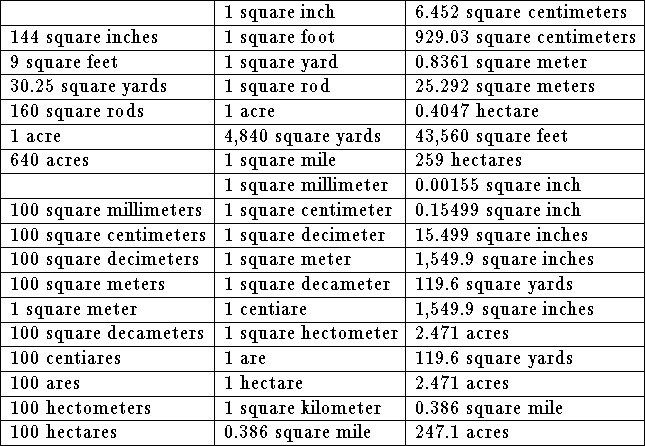Example 1: Convert 180 square inches to square feet.

Solution:

• 180 square inches can be written as 180 x 1 square inch.

• Since 1 square inch can be written as 1 inch x 1 inch,

• 180 square inches can be written 180 x 1 inch x 1 inch.

• Note that 1 inch can be written as 1 inch x 1 and the 1 can be written as a fraction where the numerator and the denominator are equivalent.

• Write 1 inch x 1 as 1 inch x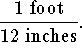• This can be further simplified to read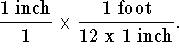• 180 square inches = 180 x 1 inch x 1 inch = 180 x 1 inch x 1 x 1 inch x 1

=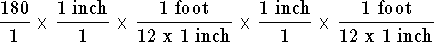• Multiply the fractions.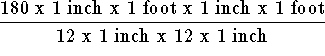• Rearrange the numerator and rearrange the denominator.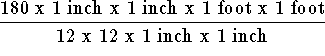• Rewrite as five fractions where as many fractions as possible have an equivalent value of 1.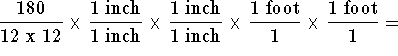1.25 x 1 x 1 x 1 foot x 1 foot

• Note: 1 foot x 1 foot is the same as one foot squared is the same as one square foot.

• The answer is 1.25 square feet.

• Since 1 square foot is equivalent to 144 square inches, the fraction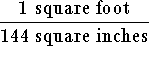is equivalent to 1.

Therefore, another way to work the problem is as follows: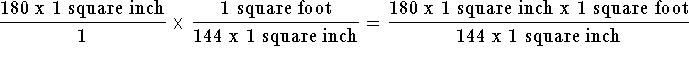=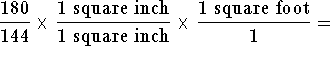1.25 x 1 x 1 square foot = 1.25 square feet

If you would like to review another example, click on Example.

If you would like to work some problems, click on Problems.[Units of Conversion]S.O.S MATHematics home page

Do you need more help? Please post your question on our S.O.S. Mathematics CyberBoard.Author: Nancy Marcus